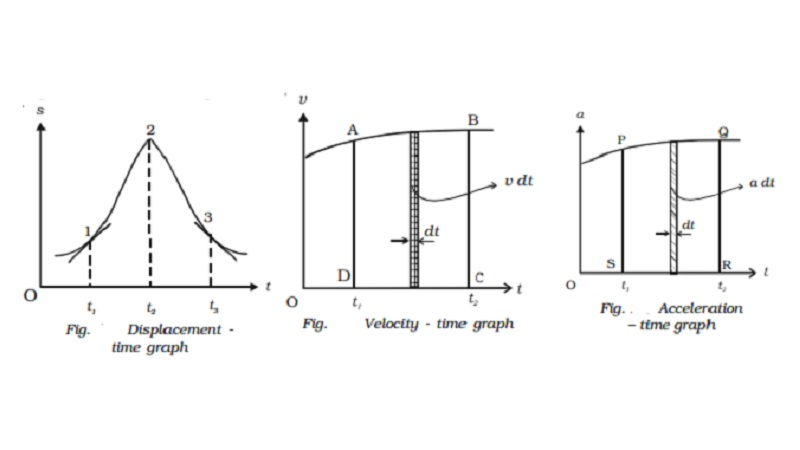Home | | Physics | | Physics | Graphical representations - displacement, velocity,acceleration with time graph

# Graphical representations - displacement, velocity,acceleration with time graphThe graphs provide a convenient method to present pictorially, the basic informations about a variety of events. Line graphs are used to show the relation of one quantity say displacement or velocity with another quantity such as time. If the displacement, velocity and acceleration of a particle are plotted with respect to time, they are known as,

Graphical representations

The graphs provide a convenient method to present pictorially, the basic informations about a variety of events. Line graphs are used to show the relation of one quantity say displacement or velocity with another quantity such as time.

If the displacement, velocity and acceleration of a particle are plotted with respect to time, they are known as,

1.  displacement ? time graph (s - t graph)

2.    velocity ? time graph (v - t graph)

3.      acceleration ? time graph (a - t graph)

Displacement ? time graph

When the displacement of the particle is plotted as a function of time, it is  displacement - time graph.

As v = ds/dt , the slope of the s - t graph at any instant gives the velocity of the particle at that instant. In Fig. 2.4 the particle at time t1, has a positive velocity, at time t2, has zero velocity and at time t3, has negative velocity.Velocity ? time graph

When the velocity of the particle is plotted as a function of time, it is velocity-time graph.

As a = dv/dt , the slope of the v ? t curve at any instant gives the acceleration of the particle (Fig. 2.5).

But v=ds/dt or

Ds = v dt

If the displacements are s1 and s2 in times t1 and t2 then

12ds = ∫ t1t2 vdt

S2-s1 = ∫t1t2vdt = area ABCD

The area under the v ? t curve, between the given intervals of time, gives the change in displacement or the distance travelled by the particle during the same interval.Acceleration ? time graph

When the acceleration is plotted as a function of time, it is acceleration ? time graph (Fig. 2.6).

A=dv/dt

If the velocities are v1 and v2 at times t1 and t2 respectively, then

v1v2 dv = ∫t1t2 a dt

V2-v1 = ∫t1t2adt =  area PQRS

The area under the a ? t curve, between the given intervals of time, gives the change in velocity of the particle during the same interval. If the graph is parallel to the time axis, the body moves with constant

acceleration.

Study Material, Lecturing Notes, Assignment, Reference, Wiki description explanation, brief detail

Related Topics